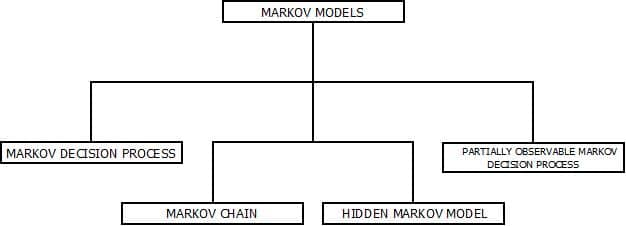# HIDDEN MARKOV MODEL

Hidden Markov Model | Artificial Intelligence Tutorial | Minigranth

# Hidden Markov Model(HMM) : Introduction

• Hidden Markov Model is an temporal probabilistic model for which a single discontinuous random variable determines all the states of the system.
• It means that, possible values of variable = Possible states in the system.
• For example: Sunlight can be the variable and sun can be the only possible state.
• The structure of Hidden Markov model is restricted to the fact that basic algorithms can be implemented using matrix representations.

### Hidden Markov Model : The Concept

• In Hidden Markov Model, every individual states has limited number of transitions and emissions.
• Probability is assigned for each transition between states.
• Hence, the past states are totally independent of future states.
• The fact that HMM is called hidden because of its ability of being a memory less process i.e. its future and past states are not dependent on each other.
•  Since, HMM is rich in mathematical structure it can be implemented for practical applications.
• This can be achieved on two algorithms called as:
1. Forward Algorithm.
2. Backward Algorithm.

### Applications : Hidden Markov Model

• Speech Recognition.
• Gesture Recognition.
• Language Recognition.
• Motion Sensing and Analysis.
• Protein Folding.

### Markov Model

• Markov model is an un-precised model that is used in the systems that does not have any fixed patterns of occurrence  i.e. randomly changing systems.
• Markov model is based upon the fact of having a random probability distribution or pattern that may be analysed statistically but cannot be predicted precisely.
• In Markov model, it is assumed that the future states only depends upon the current states and not the previously occurred states.
• There are four common Markov models out of which the most commonly used is the hidden Markov model.Types of Markov Model